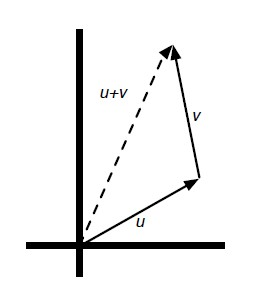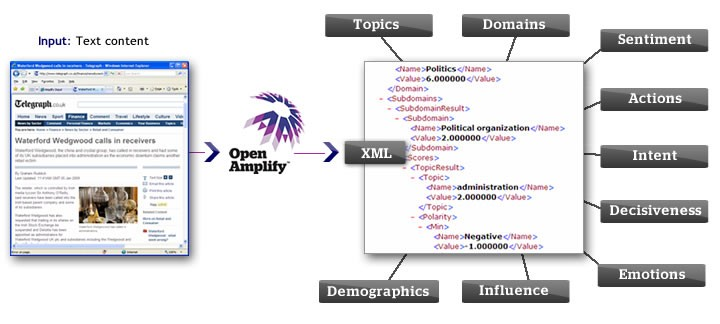# Linear Algebra - (Dot|Scalar|Inner) Product of two vectors

A dot Product is the multiplication of two two equal-length sequences of numbers (usually coordinate vectors) that produce a scalar (single number)

Dot-product is also known as:

• scalar product
• or sometimes inner product in the context of Euclidean space,

The name:

## Representation

For two vectors $u$ and $v$ , you may see the dot product of $u$ and $v$ represented as: $$u \cdot v$$ or: $$u^\top v$$

## Definition

This operation can be defined either algebraically or geometrically.

### Algebraic

The sum of the products of the corresponding entries of the two sequences of numbers.

Dot-product of two D-vectors is sum of product of corresponding entries:

$$u · v = \sum_{k \in D}{u_k.v_k}$$

### Geometric

The product of the Euclidean magnitudes of the two vectors and the cosine of the angle between them.

$$u.v = ||u|| ||v|| \cos \theta$$ where:

• cos is the cosine
• and $||u||$ and $||v||$ are the norm of each vector

## Example

### Algebraic

• For traditional vectors u = [u1, . . . , un] and v = [v1, . . . , vn]:

$$u · v = u_1.v_1 + u_2.v_2 + \dots + u_n.v_n$$

• Dot-product of [1, 1, 1, 1, 1] and [10, 20, 0, 40,-100]
   1   1    1    1       1
x 10   20   0   40    -100
= 10 + 20 + 0 + 40 + (-100) = -30


## Python Code

### Algebraic

def list_dot(u, v): return sum([a*b for (a,b) in zip(u,v)])


## Application

### A quantity and price vector

quantity = {"lemon": 2, "orange" : 3}
price = {"lemon": 1.5, "orange" : 2}
quantity x price = 2x1.5 + 2x3 = 9


### Similarity

• When the value of the dot product is positive of maximal the two vectors tend to agree.
• When the value of the two vectors is negative of minimal the two vectors tend to disagree.

Example: similarity in voting system: With a voting system (neutral:0, for:1, against:-1) if both values of two votes are 1, the corresponding term in the sum is 1. If both values are -1, the corresponding term in the sum is also 1. Thus a term in the sum that is 1 indicates agreement.

## Documentation / Reference

Discover MoreData Mining - (Stochastic) Gradient descent (SGD)

Gradient descent can be used to train various kinds of regression and classification models. It's an iterative process and therefore is well suited for map reduce process. The gradient descent update...Linear Algebra - Vector Vector Operations

Vector Vector Operations: (also known as addition or substraction) (Scaling) Using operator overloading, you can compute this operations with the following syntax. Operation Syntax...Natural Language - Document (Cosine) Similarity

Cosine similarity applied to document similarity. Each document becomes a vector in some high dimensional space. To compare two documents we compute the cosine of the angle between their two document...Python - NumPy

NumPy is a Python library for working with arrays. NumPy provides abstractions that make it easy to treat these underlying arrays as vectors and matrices....What is the Cosine Similarity or Cosine Distance? (Measure of Angle)

The cosine similarity (or cosine distance) is a distance that measures the angle between two vectors, normalized by magnitude. You just divide the dot product by the magnitude of the two vectors. ...